# Frank solutions for Class 9 Maths ICSE chapter 15 - Mid-point and Intercept Theorems [Latest edition]

#### Chapters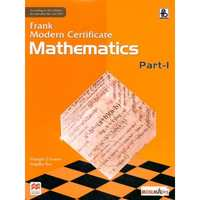## Chapter 15: Mid-point and Intercept Theorems

Exercise 15.1Exercise 15.2
Exercise 15.1

### Frank solutions for Class 9 Maths ICSE Chapter 15 Mid-point and Intercept Theorems Exercise 15.1

Exercise 15.1 | Q 1

In ΔABC, D is the mid-point of AB and E is the mid-point of BC.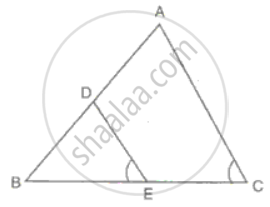Calculate:
(i) DE, if AC = 8.6 cm
(ii) ∠DEB, if ∠ACB = 72°

Exercise 15.1 | Q 2

In ΔABC, AB = 12 cm and AC = 9 cm. If M is the mid-point of AB and a straight line through M parallel to AC cuts BC in N, what is the length of MN?

Exercise 15.1 | Q 3.1

In ΔABC, D, E, F are the midpoints of BC, CA and AB respectively. Find FE, if BC = 14 cm

Exercise 15.1 | Q 3.2

In ΔABC, D, E, F are the midpoints of BC, CA and AB respectively. Find DE, if AB = 8 cm

Exercise 15.1 | Q 3.3

In ΔABC, D, E, F are the midpoints of BC, CA and AB respectively. Find ∠FDB if ∠ACB = 115°.

Exercise 15.1 | Q 4

In parallelogram PQRS, L is mid-point of side SR and SN is drawn parallel to LQ which meets RQ produced at N and cuts side PQ at M. Prove that M is the mid-point of PQ.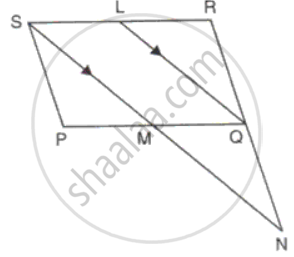Exercise 15.1 | Q 5.1

In ΔABC, BE and CF are medians. P is a point on BE produced such that BE = EP and Q is a point on CF produced such that CF = FQ. Prove that: QAP is a straight line.

Exercise 15.1 | Q 5.2

In ΔABC, BE and CF are medians. P is a point on BE produced such that BE = EP and Q is a point on CF produced such that CF = FQ. Prove that: A is the mid-point of PQ.

Exercise 15.1 | Q 6

Prove that the figure obtained by joining the mid-points of the adjacent sides of a rectangle is a rhombus.

Exercise 15.1 | Q 7

D, E and F are the mid-points of the sides AB, BC and CA of an isosceles ΔABC in which AB = BC. Prove that ΔDEF is also isosceles.

Exercise 15.1 | Q 8

Prove that the straight lines joining the mid-points of the opposite sides of a quadrilateral bisect each other.

Exercise 15.1 | Q 9

The diagonals of a quadrilateral intersect each other at right angle. Prove that the figure obtained by joining the mid-points of the adjacent sides of the quadrilateral is a rectangle.

Exercise 15.1 | Q 10

If L and M are the mid-points of AB, and DC respectively of parallelogram ABCD. Prove that segment DL and BM trisect diagonal AC.

Exercise 15.1 | Q 11

In a right-angled triangle ABC. ∠ABC = 90° and D is the midpoint of AC. Prove that BD = (1)/(2)"AC".

Exercise 15.1 | Q 12

In parallelogram ABCD, P is the mid-point of DC. Q is a point on AC such that CQ = (1)/(4)"AC". PQ produced meets BC at R. Prove that

(i) R is the mid-point of BC, and

(ii) PR = (1)/(2)"DB".

Exercise 15.1 | Q 13

In a parallelogram ABCD, M is the mid-point AC. X and Y are the points on AB and DC respectively such that AX = CY. Prove that:
(i) Triangle AXM is congruent to triangle CYM, and

(ii) XMY is a straight line.

Exercise 15.1 | Q 14

Show that the quadrilateral formed by joining the mid-points of the adjacent sides of a square is also a square.

Exercise 15.1 | Q 15.1

In the given figure, ABCD is a trapezium. P and Q are the midpoints of non-parallel side AD and BC respectively. Find: PQ, if AB = 12 cm and DC = 10 cm.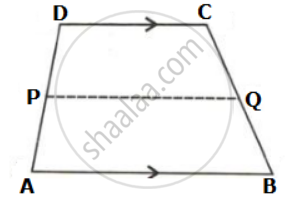Exercise 15.1 | Q 15.2

In the given figure, ABCD is a trapezium. P and Q are the midpoints of non-parallel side AD and BC respectively. Find: AB, if DC = 8 cm and PQ = 9.5 cm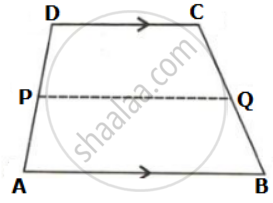Exercise 15.1 | Q 15.3

In the given figure, ABCD is a trapezium. P and Q are the midpoints of non-parallel side AD and BC respectively. Find: DC, if AB = 20 cm and PQ = 14 cm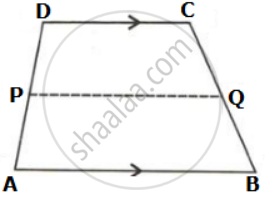Exercise 15.1 | Q 16.1

In ΔABC, P is the mid-point of BC. A line through P and parallel to CA meets AB at point Q, and a line through Q and parallel to BC meets median AP at point R. Prove that: AP = 2AR

Exercise 15.1 | Q 16.2

In ΔABC, P is the mid-point of BC. A line through P and parallel to CA meets AB at point Q, and a line through Q and parallel to BC meets median AP at point R. Prove that: BC = 4QR

Exercise 15.1 | Q 17.1

Side AC of a ABC is produced to point E so that CE = (1)/(2)"AC". D is the mid-point of BC and ED produced meets AB at F. Lines through D and C are drawn parallel to AB which meets AC at point P and EF at point R respectively. Prove that: 3DF = EF

Exercise 15.1 | Q 17.2

Side AC of a ABC is produced to point E so that CE = (1)/(2)"AC". D is the mid-point of BC and ED produced meets AB at F. Lines through D and C are drawn parallel to AB which meets AC at point P and EF at point R respectively. Prove that: 4CR = AB.

Exercise 15.1 | Q 18

AD is a median of side BC of ABC. E is the midpoint of AD. BE is joined and produced to meet AC at F. Prove that AF: AC = 1 : 3.

Exercise 15.1 | Q 19

M and N divide the side AB of ΔABC into three equal parts. Line segments MP and NQ are both parallel to BC, and meet AC in P and Q respectively. Prove that P and Q divide AC into three equal parts.

Exercise 15.1 | Q 20

ΔABC is an isosceles triangle with AB = AC. D, E and F are the mid-points of BC, AB and AC respectively. Prove that the line segment AD is perpendicular to EF and is bisected by it.

Exercise 15.1 | Q 21.1

ABCD is a parallelogram.E is the mid-point of CD and P is a point on AC such that PC = (1)/(4)"AC". EP produced meets BC at F. Prove that: F is the mid-point of BC.

Exercise 15.1 | Q 21.2

ABCD is a parallelogram.E is the mid-point of CD and P is a point on AC such that PC = (1)/(4)"AC". EP produced meets BC at F. Prove that: 2EF = BD.

Exercise 15.1 | Q 22.1

ABCD is a kite in which BC = CD, AB = AD. E, F and G are the mid-points of CD, BC and AB respectively. Prove that: ∠EFG = 90°

Exercise 15.1 | Q 22.2

ABCD is a kite in which BC = CD, AB = AD. E, F and G are the mid-points of CD, BC and AB respectively. Prove that: The line drawn through G and parallel to FE and bisects DA.

Exercise 15.1 | Q 23

In ΔABC, X is the mid-point of AB, and Y is the mid-point of AC. BY and CX are produced and meet the straight line through A parallel to BC at P and Q respectively. Prove AP = AQ.

Exercise 15.1 | Q 24.1

In ΔABC, D, E and F are the midpoints of AB, BC and AC.
Show that AE and DF bisect each other.

Exercise 15.1 | Q 24.2

In ΔABC, D, E and F are the midpoints of AB, BC and AC.
If AE and DF intersect at G, and M and N are the midpoints of GB and GC respectively, prove that DMNF is a parallelogram.

Exercise 15.2

### Frank solutions for Class 9 Maths ICSE Chapter 15 Mid-point and Intercept Theorems Exercise 15.2

Exercise 15.2 | Q 1.1

In a parallelogram ABCD, E and F are the midpoints of the sides AB and CD respectively. The line segments AF and BF meet the line segments DE and CE at points G and H respectively Prove that: ΔGEA ≅ ΔGFD

Exercise 15.2 | Q 1.2

In a parallelogram ABCD, E and F are the midpoints of the sides AB and CD respectively. The line segments AF and BF meet the line segments DE and CE at points G and H respectively Prove that: ΔHEB ≅ ΔHFC

Exercise 15.2 | Q 1.3

In a parallelogram ABCD, E and F are the midpoints of the sides AB and CD respectively. The line segments AF and BF meet the line segments DE and CE at points G and H respectively Prove that: EGFH is a parallelogram.

Exercise 15.2 | Q 2.1

In ΔABC, the medians BE and CD are produced to the points P and Q respectively such that BE = EP and CD = DQ. Prove that: Q A and P are collinear.

Exercise 15.2 | Q 2.2

In ΔABC, the medians BE and CD are produced to the points P and Q respectively such that BE = EP and CD = DQ. Prove that: A is the mid-point of PQ.

Exercise 15.2 | Q 3

In AABC, D and E are two points on the side AB such that AD = DE = EB. Through D and E, lines are drawn parallel to BC which meet the side AC at points F and G respectively. Through F and G, lines are drawn parallel to AB which meet the side BC at points M and N respectively. Prove that BM = MN = NC.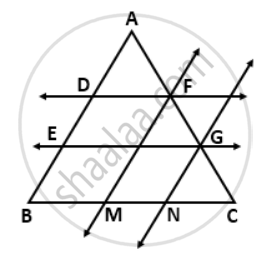Exercise 15.2 | Q 4

In the given figure, the lines l, m and n are parallel to each other. D is the midpoint of CE. Find: a. BC, b. EF, c. CG and d. BD.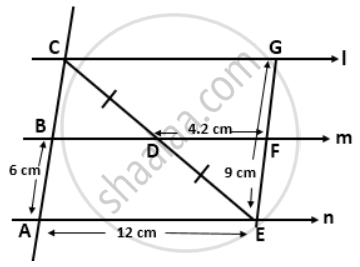Exercise 15.2 | Q 5

The diagonals AC and BD of a quadrilateral ABCD intersect at right angles. Prove that the quadrilateral formed by joining the midpoints of quadrilateral ABCD is a rectangle.

Exercise 15.2 | Q 6

In ΔABC, D and E are the midpoints of the sides AB and AC respectively. F is any point on the side BC. If DE intersects AF at P show that DP = PE.

Exercise 15.2 | Q 7

In ΔABC, D and E are the midpoints of the sides AB and BC respectively. F is any point on the side AC. Also, EF is parallel to AB. Prove that BFED is a parallelogram.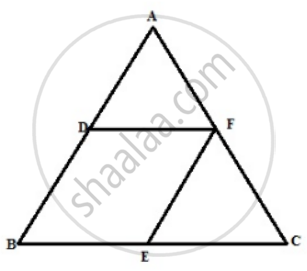Remark: Figure is incorrect in Question

Exercise 15.2 | Q 8.1

In the given figure, PS = 3RS. M is the midpoint of QR. If TR || MN || QP, then prove that: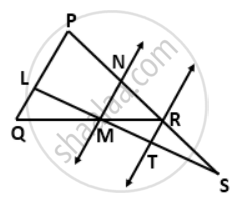ST = (1)/(3)"LS"

Exercise 15.2 | Q 8.2

In the given figure, PS = 3RS. M is the midpoint of QR. If TR || MN || QP, then prove that:RT = (1)/(3)"PQ"

Exercise 15.2 | Q 9

In the given figure, T is the midpoint of QR. Side PR of ΔPQR is extended to S such that R divides PS in the ratio 2:1. TV and WR are drawn parallel to PQ. Prove that T divides SU in the ratio 2:1 and WR = (1)/(4)"PQ".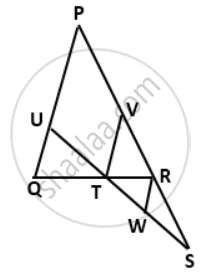## Chapter 15: Mid-point and Intercept Theorems

Exercise 15.1Exercise 15.2## Frank solutions for Class 9 Maths ICSE chapter 15 - Mid-point and Intercept Theorems

Frank solutions for Class 9 Maths ICSE chapter 15 (Mid-point and Intercept Theorems) include all questions with solution and detail explanation. This will clear students doubts about any question and improve application skills while preparing for board exams. The detailed, step-by-step solutions will help you understand the concepts better and clear your confusions, if any. Shaalaa.com has the CISCE Class 9 Maths ICSE solutions in a manner that help students grasp basic concepts better and faster.

Further, we at Shaalaa.com provide such solutions so that students can prepare for written exams. Frank textbook solutions can be a core help for self-study and acts as a perfect self-help guidance for students.

Concepts covered in Class 9 Maths ICSE chapter 15 Mid-point and Intercept Theorems are The Mid-point Theorem, Equal Intercept Theorem.

Using Frank Class 9 solutions Mid-point and Intercept Theorems exercise by students are an easy way to prepare for the exams, as they involve solutions arranged chapter-wise also page wise. The questions involved in Frank Solutions are important questions that can be asked in the final exam. Maximum students of CISCE Class 9 prefer Frank Textbook Solutions to score more in exam.

Get the free view of chapter 15 Mid-point and Intercept Theorems Class 9 extra questions for Class 9 Maths ICSE and can use Shaalaa.com to keep it handy for your exam preparation